# High School Physics : Understanding Significant Figures

## Example Questions

← Previous 1

### Example Question #1 : Understanding Significant Figures

How many significant figures should be kept when multiplying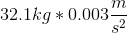?

5

1

2

0

3

3

Explanation:

Always keep the least number of significant figures. Two types of figures can be significant: non-zero numbers and zeroes that come after the demical place.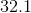has 3 significant figures while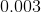also has 3.

### Example Question #2 : Understanding Significant Figures

How many significant figures are in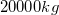?

One

Five

Seven

Four

Zero

One

Explanation:

There are two categories that count as significant figures: non-zero numbers before the decimal point and everything after the decimal point. Since the number given is, there is only one non-zero number before the decimal point.

This number could have been rounded from any variation past the first digit, and we cannot assume that it is exact. To write this number in exact terms, we must use scientific notation or a decimal.

### Example Question #1 : Understanding Significant Figures

How many significant figures should be in in the answer to the calculation?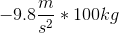Two

Five

Three

One

Four

One

Explanation:

There are two categories that count as significant figures: non-zero numbers before the decimal point and everything after the decimal point.

When performing any operation between two numbers with different significant figures, always base it off the smallest number of significant figures. In this case,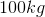has only one significant figure; therefore our answer should also have only one significant figure.

### Example Question #71 : Introductory Principles

How many significant figures should be in the answer to the calculation?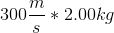Three

Zero

Six

One

Two

One

Explanation:

Your answer should have the same number of significant figures as the term with the fewest significant figures. There are only two categories that qualify as significant figures: any terms after the decimal point and non-zero digits before the decimal.

In this problem,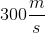has the fewest number of significant figures: only one.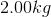has three significant figures, but its precision is diminished by multiplying by a less precise term.

### Example Question #5 : Understanding Significant Figures

Solve the expression with the proper number of significant figures.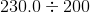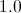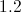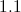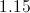Explanation:

When multiplying or dividing, the answer must have the same number of significant figures as the term with the fewest significant figures in the problem. In this question, the one term () has only significant figure, and the other term (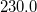) has four. Our final answer must match the term with the fewest significant figures: one. It is important to remember that zeroes after a decimal are significant figures, and determine the precision of a value. Zeroes before a decimal, such as in, simply serve as place holders, and are no considered significant unless there is a decimal or scientific notation to clarify the term.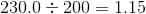Round to the ones place to get an answer with only one significant figure.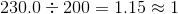### Example Question #72 : Introductory Principles

Solve the expression with the proper number of significant figures.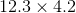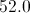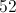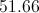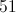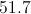Explanation:

When multiplying or dividing, the answer must have the same number of significant figures as the term with the fewest significant figures in the problem. In this question, the one term (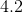) has two significant figures, and the other term (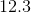) has three. Our final answer must match the term with the fewest significant figures: two.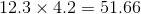Round to the tens place to get an answer with only two significant figures.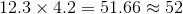### Example Question #1 : Understanding Significant Figures

How many significant figures should be in the solution to the given equation?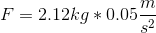Six

Zero

One

Three

Two

One

Explanation:

Leading and trailing zeroes are not considered significant. To make this problem simpler, we may want to re-write the equation in scientific notation.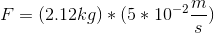The first term still has three digits, all of which are significant. The second term, however, has been reduced to only one digit. Our term with the fewest significant figures determines how many significant figures should be in the solution. In this case, there would only be one significant figure in the solution.

### Example Question #8 : Understanding Significant Figures

Which of the following numbers contains the greatest number of significant figures?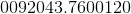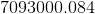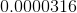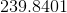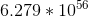Explanation:

Significant figures are used to round scientific calculations in order to preserve precision. Every initial measurement will carry some degree of uncertainty or variation. It is important to carry that uncertainty to the final calculation, instead of unintentionally assigning greater certainty to the given values.

When determining significant figures, any zeroes that precede the first non-zero digit are considered insignificant and any zeroes that follow the final non-zero digit are considered insignificant. Any and all non-zero digits are significant. Sometimes it can help to write a number in scientific notation to identify significant figures.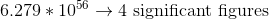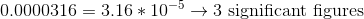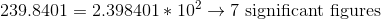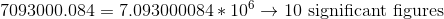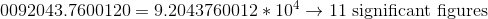### Example Question #9 : Understanding Significant Figures

Which of the following answers does not have the correct number of significant figures associated with it?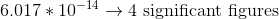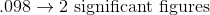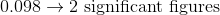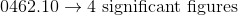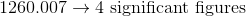Explanation:

Significant figures are used to round scientific calculations in order to preserve precision. Every initial measurement will carry some degree of uncertainty or variation. It is important to carry that uncertainty to the final calculation, instead of unintentionally assigning greater certainty to the given values.

When determining significant figures, any zeroes that precede the first non-zero digit are considered insignificant and any zeroes that follow the final non-zero digit are considered insignificant. Any and all non-zero digits are significant. Sometimes it can help to write a number in scientific notation to identify significant figures.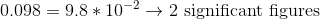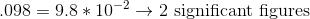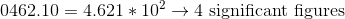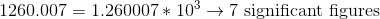### Example Question #10 : Understanding Significant Figures

Which of the following answers has the correct number of significant figures associated with it?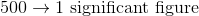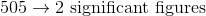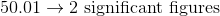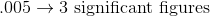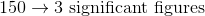Explanation:

Significant figures are used to round scientific calculations in order to preserve precision. Every initial measurement will carry some degree of uncertainty or variation. It is important to carry that uncertainty to the final calculation, instead of unintentionally assigning greater certainty to the given values.

When determining significant figures, any zeroes that precede the first non-zero digit are considered insignificant and any zeroes that follow the final non-zero digit are considered insignificant. Any and all non-zero digits are significant. Sometimes it can help to write a number in scientific notation to identify significant figures.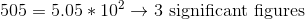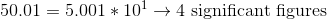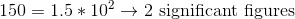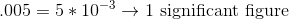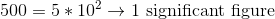← Previous 1